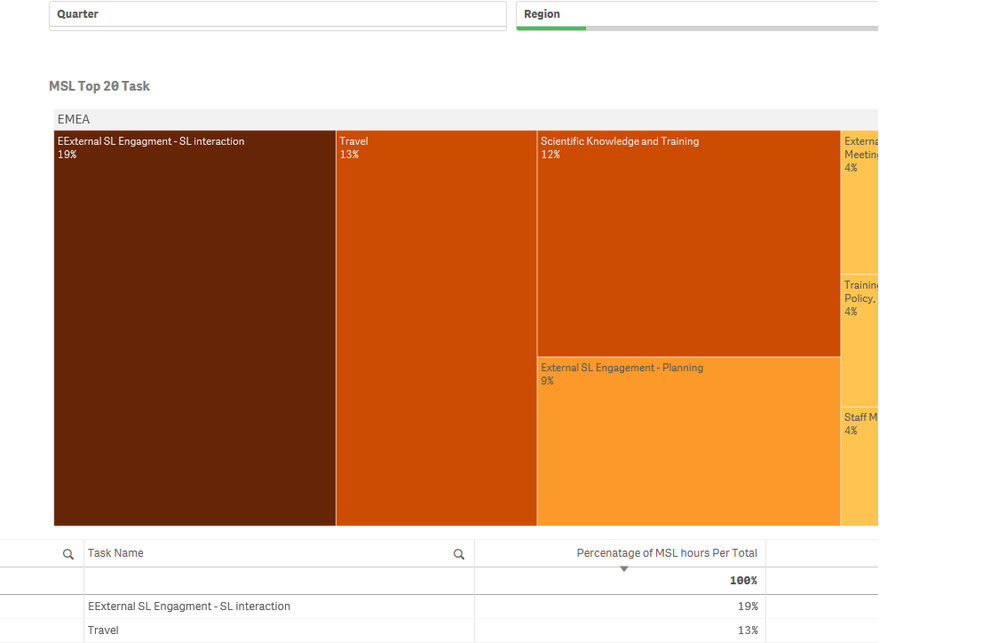# New to Qlik Sense

Discussion board where members can get started with Qlik Sense.

Announcements
QlikWorld, June 24-25, 2020. Free virtual event for DI and DA gurus. Register Now
cancel
Showing results for
Did you mean:
HighlightedCreator II

## Calculating Percentage of Total By Regionin Qliksense

Hi,

I have to calculate percentage of MSL hours by total for each region, say in a Table or Treemap.

1. Dimension- Region,

2. Dimension -Task (Fixed to Top 20 ).

3. Measure is : =(sum({<FM={'MSL'}>}Q1hours)+sum({<FM={'MSL'}>}Q2hours)+sum({<FM={'MSL'}>}Q3hours)+sum({<FM={'MSL'}>}Q4hours)) /
(sum(total{<FM={'MSL'}>}Q1hours)+sum(total{<FM ={'MSL'}>}Q2hours)+sum(total{<FM ={'MSL'}>}Q3hours)+ sum(total{<FM ={'MSL'}>}Q4hours))

This gives me a decimal values and I convert it to Percentage.

I need to add Region also in code not sure how to do.

With above code I got below Screen ShotWhen I select Region in dashboard  The Region as EMEA , the shot comes like below and  that is what I want to be displayed.How should I display the data without selecting Region as filter in dashboard but rather as code in measure.

Regards,

Niha

1 Solution

Accepted Solutions
HighlightedPartner

## Re: Calculating Percentage of Total By Regionin Qliksense

Try this measure

=(sum({<FM={'MSL'}>}Q1hours)+sum({<FM={'MSL'}>}Q2hours)+sum({<FM={'MSL'}>}Q3hours)+sum({<FM={'MSL'}>}Q4hours)) /
(sum(total <Region> {<FM={'MSL'}>}Q1hours)+sum(total <Region>{<FM ={'MSL'}>}Q2hours)+sum(total<Region>{<FM ={'MSL'}>}Q3hours)+ sum(total<Region>{<FM ={'MSL'}>}Q4hours))

Plees ekskuse my Swenglish and or Norweglish spelling misstakes
2 Replies
HighlightedPartner

## Re: Calculating Percentage of Total By Regionin Qliksense

Try this measure

=(sum({<FM={'MSL'}>}Q1hours)+sum({<FM={'MSL'}>}Q2hours)+sum({<FM={'MSL'}>}Q3hours)+sum({<FM={'MSL'}>}Q4hours)) /
(sum(total <Region> {<FM={'MSL'}>}Q1hours)+sum(total <Region>{<FM ={'MSL'}>}Q2hours)+sum(total<Region>{<FM ={'MSL'}>}Q3hours)+ sum(total<Region>{<FM ={'MSL'}>}Q4hours))

Plees ekskuse my Swenglish and or Norweglish spelling misstakes
HighlightedCreator II

Worked!